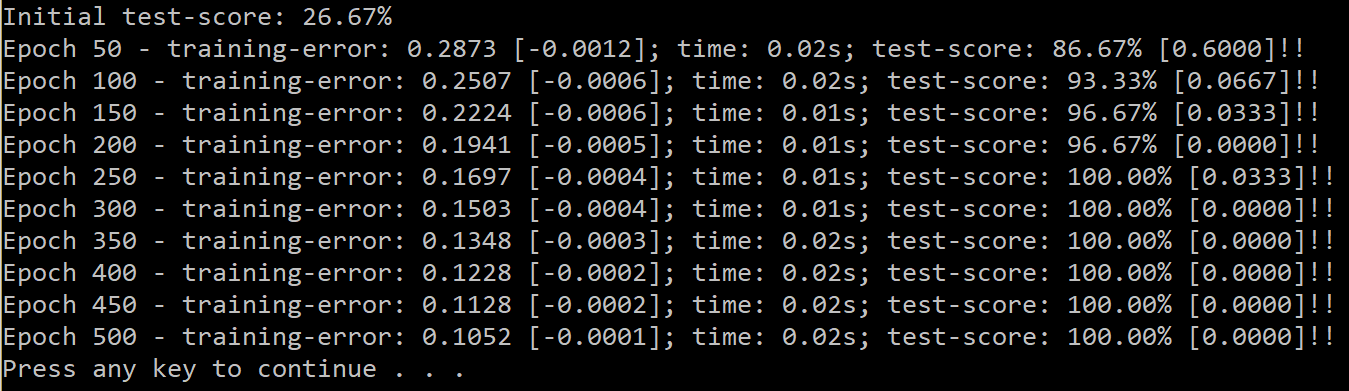Bright Wire is designed to be easily extended. This tutorial shows how to create and use a SELU activation function that can be used to train deep feed forward neural networks along with batch normalisation.

Motivation

New research papers are published every day with potentially interesting new techniques to try. Bright Wire is designed to be a solid foundation that can be easily extended with new components in a modular way. This tutorial demonstrates extending Bright Wire with a SELU activation function as described in Self-Normalizing Neural Networks. This activation function is claimed to introduce self normalizing properties to help train deep (many layers of) feed forward neural networks. More specifically, the gradient from the activation function will not vanish (as with RELU) as the layers get deeper.

Custom Activation Function

Bright Wire works as a graph of nodes that receive matrices or tensors from their parent(s). Nodes calculate new results to pass onto to their children and also potentially receive error signals which flow backwards along the graph. Nodes can "learn" improved parameters in response to the error signals and can update the error signal (back propagation) so that prior nodes learn improved parameters themselves.

So, to add a new type of activation function we need to create a new type of node (that implements INode) and instantiate it into our graph.

There is a convenient NodeBase class that makes implementing this interface easy. All we need to do is implement the ExecuteForward function, which gets passed an IContext that contains the current state of the graph.

From the context we can access the signal as a matrix and apply the SELU activation into a new matrix that will become the output from the node. Because the error signal will need to be transformed to apply the SELU back-propagation, we pass a back-propagation creation function. This is lazily created to avoid creating unnecessary objects during non-training graph execution.

During training, the back-propagation class is instantiated and used to back-propagate the SELU activation. This involves replacing the error signal with a new error matrix (using the gradient/derivative of the activation).

class SeluActivation : NodeBase
{
const float ALPHA = 1.6732632423543772848170429916717f;
const float SCALE = 1.0507009873554804934193349852946f;

/// <summary>
/// Backpropagation of SELU activation
/// </summary>
class Backpropagation : SingleBackpropagationBase<SeluActivation>
{
public Backpropagation(SeluActivation source) : base(source) { }

protected override IGraphData _Backpropagate(INode fromNode, IGraphData errorSignal, IContext context, IReadOnlyList<INode> parents)
{
var matrix = errorSignal.GetMatrix().AsIndexable();
var delta = context.LinearAlgebraProvider.CreateMatrix(matrix.RowCount, matrix.ColumnCount, (i, j) => {
var x = matrix[i, j];
if(x >= 0)
return SCALE;
return SCALE * ALPHA * BoundMath.Exp(x);
});
return errorSignal.ReplaceWith(delta);
}
}

public SeluActivation(string name = null) : base(name) {}

public override void ExecuteForward(IContext context)
{
var matrix = context.Data.GetMatrix().AsIndexable();
var output = context.LinearAlgebraProvider.CreateMatrix(matrix.RowCount, matrix.ColumnCount, (i, j) => {
var x = matrix[i, j];
if (x >= 0)
return SCALE * x;
return SCALE * (ALPHA * BoundMath.Exp(x) - ALPHA);
});
_AddNextGraphAction(context, context.Data.ReplaceWith(output), () => new Backpropagation(this));
}
}

Batch Normalisation

At least on this data-set, SELU seemed to work best when the input was normalized before activation (which is disappointing given that the "self normalizing neural networks" as described in the paper are supposed not to need additional normalization).

It's easy to normalize the input to a neural network, we just adjust the training and test data so that each feature follows a standard distribution (Normalise(NormalisationType.Standard) in Bright Wire). To normalize the output of a feed forward layer we can introduce a batch normalization layer after each feed forward layer (and before the activation).

A batch normalization layer learns the distribution of its input and outputs a normalized version of each batch based on the mean and standard deviation that it has observed across all batches. (It also learns two parameters that can scale and shift the output if that seems beneficial).

We add a batch normalization layer before each SELU activation in the code below. This neural network has seven feed forward layers and uses the custom activation function above and batch normalization before each activation. The final activation function is soft max and the error metric is OneHotEncoding which considers the highest output to be 1 and every other output to be zero, which it then compares against the expected one hot encoded training data.

graph.Connect(engine)
;

Results

In this case SELU was able to train a deep neural network faster and more successfully than RELU (or even leaky RELU) and quickly reached a perfect accuracy on this admittedly toy problem. As discussed, SELU needed batch normalization to train successfully. But an observed benefit at least in this case is that training was productive even with large batch sizes, which seemed like a promising result.

So it's clear that there are many possible approaches in machine learning and deep feed forward networks with SELU is indeed a tool worth considering.Source Code

View the complete source on GitHub.